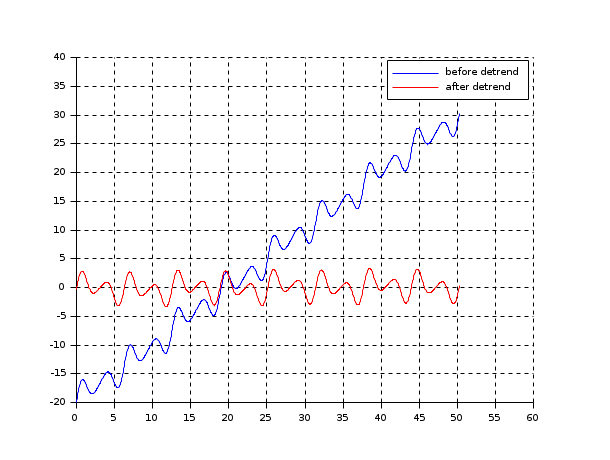## Linear Detrend

Takes a time series dataset, fits a linear trend to the data, and returns the dataset with the linear trend subtracted out.## Inputs

### JSON Format

You must set either `uniformlySpaced` or `variablySpaced` json values, but not both.

``````{
"uniformlySpaced": Double[],
"variablySpaced": Double[][]
}
``````

uniformly spaced

``````{"uniformlySpaced": [1,2,3,4,3,4,6,7,5,4,2,1,1]}
``````

variably spaced

``````{"variablySpaced": [[1,2,3,4,5,6,6.5,7,8.5,9,10,11,12],[1,2,3,4,3,4,6,7,5,4,2,1,1]]}
``````
• uniformlySpaced - (pick one) - dataset is a 1D array filled with uniformly spaced y coordinates, assumes all datapoints are uniformly spaced in the X axis (IE: input[y] where `dx(1) = dx(n)`)
• variablySpaced - (pick one) - Dataset is a 2D array where with the first axis X and second Y (IE: `input[X][Y]`). both arrays must be the same size.

## Array Format

``````[
first: Double[],
second: Double[]
]

``````

uniformly spaced

``````[[1,2,3,4,3,4,6,7,5,4,2,1,1]]
``````

variably spaced

``````[[1,2,4,8,16,32,64,128,256,512],[1,2,3,4,3,4,6,7,5,4]]
``````
• first - (required) - if second is not defined, this field defines the dataset's Y axis, and assumes that all datapoints are uniformly spaced in the X axis (IE: `dx(1) = dx(n)`)
• second - (optional) - if defined, first becomes the X axis coordinates for each datapoint, and second becomes the Y axis for each datapoint.

## Outputs

This algorithm output's in the same format it recieved input: if you passed it JSON data it returns JSON data, if you passed it array data it returns array data, etc.

## JSON Format

``````{"output": [
-2.373626373626374,
-1.3626373626373631,
-0.3516483516483522,
0.6593406593406588,
-0.3296703296703303,
0.6813186813186807,
2.6923076923076916,
3.7032967032967026,
1.7142857142857135,
0.7252747252747245,
-1.2637362637362641,
-2.252747252747253,
-2.241758241758242
]}
``````
• output - The input datset with any linear trend removed.

## Array Format

``````[
-2.373626373626374,
-1.3626373626373631,
-0.3516483516483522,
0.6593406593406588,
-0.3296703296703303,
0.6813186813186807,
2.6923076923076916,
3.7032967032967026,
1.7142857142857135,
0.7252747252747245,
-1.2637362637362641,
-2.252747252747253,
-2.241758241758242
]
``````
• output - The input datset with any linear trend removed.

Contents